Resources by Strand

Strand: MATHEMATICAL PRACTICES (1.MP)
The Standards for Mathematical Practice in first grade describe mathematical habits of mind that teachers should seek to develop in their students. Students become mathematically proficient in engaging with mathematical content and concepts as they learn, experience, and apply these skills and attitudes (Standards 1.MP. 1-8).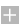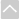Strand: OPERATIONS AND ALGEBRAIC THINKING (1.OA)
Represent and solve problems involving addition and subtraction within 20 (Standards 1–2, 5–6).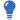Core Guide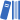OER Curriculum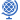Instructional TasksReview Activitiy

Standard 1.OA.1
Use addition and subtraction within 20 to solve word problems involving situations of adding to, taking from, putting together, taking apart, and comparing, with unknowns in all positions. For example, use objects, drawings, and equations with a symbol for the unknown number to represent the problem.

Standard 1.OA.2
Solve word problems that call for addition of three whole numbers whose sum is less than or equal to 20. For example, use objects, drawings, and equations with a symbol for the unknown number to represent the problem.

Understand and apply properties of operations and the relationship between addition and subtraction (Standards 1.OA.3–4).Core GuideOER CurriculumInstructional TasksReview Activitiy

Standard 1.OA.3
Apply properties of operations as strategies to add and subtract. For example: If 8 + 3 = 11 is known, then 3 + 8 = 11 is also known. (Commutative property of addition.) To add 2 + 6 + 4, the second two numbers can be added to make a ten, so 2 + 6 + 4 = 2 + 10 =12. (Associative property of addition.) First grade students need not use formal terms for these properties.

Standard 1.OA.4
Understand subtraction as an unknown-addend problem. For example, subtract 10 - 8 by finding the number that makes 10 when added to 8.

Represent and solve problems involving addition and subtraction within 20 (Standards 1–2, 5–6).Core GuideOER CurriculumInstructional TasksReview Activitiy

Standard 1.OA.5
Relate counting to addition and subtraction. For example, by counting on 2 to add 2.

Standard 1.OA.6

1. Use strategies such as counting on; making ten (for example, 8 + 6 = 8 + 2 + 4 = 10 + 4 = 14); decomposing a number leading to a ten (for example, 13 - 4 = 13 - 3 - 1 = 10 - 1 = 9); using the relationship between addition and subtraction (for example, knowing that 8 + 4 = 12, one knows 12 - 8 = 4); and creating equivalent but easier or known sums (for example, adding 6 + 7 by creating the known equivalent 6 + 6 + 1 = 12 + 1 = 13).
2. By the end of Grade 1, demonstrate fluency for addition and subtraction within 10.
Work with addition and subtraction equations (Standards 1.OA.7–8).Core GuideOER CurriculumInstructional Tasks

Standard 1.OA.7
Understand the meaning of the equal sign, and determine whether equations involving addition and subtraction are true or false. For example, which of the following equations are true and which are false? 6 = 6, 7 = 8 - 1, 5 + 2 = 2 + 5, 4 + 1 = 5 + 2.

Standard 1.OA.8
Determine the unknown whole number in an addition or subtraction equation relating three whole numbers. For example, determine the unknown number that makes the equation true in each of the equations 8 + ? = 11, 5 = ? - 3, 6 + 6 = ?Strand: NUMBER AND OPERATIONS IN BASE TEN (1.NBT)
Extend the counting sequence (Standard 1.NBT.1).Core GuideOER CurriculumReview Activitiy

Standard 1.NBT.1
Count to 120, starting at any number less than 120. In this range, read and write numerals and represent a number of objects with a written numeral.

Understand place value (Standards 1.NBT.2–3).Core GuideOER CurriculumInstructional TasksReview Activitiy

Standard 1.NBT.2
Understand that the two digits of a two-digit number represent amounts of tens and ones. Understand the following as special cases:

1. 10 can be thought of as a bundle of ten ones, called a "ten."
2. The numbers from 11 to 19 are composed of a ten and one, two, three, four, five, six, seven, eight, or nine ones.
3. The numbers 10, 20, 30, 40, 50, 60, 70, 80, 90 refer to one, two, three, four, five, six, seven, eight, or nine tens (and 0 ones).

Standard 1.NBT.3
Compare two two-digit numbers based on meanings of the tens and ones digits, recording the results of comparisons with the symbols >, =, and <.

Use place value understanding and properties of operations to add and subtract (Standards 1.NBT.4–6).Core GuideOER CurriculumInstructional Tasks

Standard 1.NBT.4
Add within 100, including adding a two-digit number and a one-digit number, and adding a two-digit number and a multiple of 10, using concrete models or drawings and strategies based on place value, properties of operations, and/or the relationship between addition and subtraction; relate the strategy to a written method and explain the reasoning used. Understand that in adding two-digit numbers, one adds tens to tens and ones to ones, and that it is sometimes necessary to compose a ten.

Standard 1.NBT.5
Given a two-digit number, mentally find 10 more or 10 less than the number, without having to count; explain the reasoning used.

Standard 1.NBT.6
Subtract multiples of 10 in the range 10-90 from multiples of 10 in the range 10-90 (positive or zero differences), using concrete models or drawings and strategies based on place value, properties of operations, and/or the relationship between addition and subtraction; relate the strategy to a written method and explain the reasoning used.Strand: MEASUREMENT AND DATA (1.MD.)
Measure lengths indirectly and by iterating length units (Standards 1.MD.1–2).Core GuideOER CurriculumInstructional TasksReview Activitiy

Standard 1.MD.1
Order three objects by length; compare the lengths of two objects indirectly by using a third object.

Standard 1.MD.2
Express the length of an object as a whole number of length units, by laying multiple copies of a shorter object (the length unit) end to end; understand that the length measurement of an object is the number of same-size length units that span it with no gaps or overlaps. Limit to contexts where the object being measured is spanned by a whole number of length units with no gaps or overlaps.

Tell and write time (Standard 1.MD.3).Core GuideOER CurriculumInstructional TasksReview Activitiy

Standard 1.MD.3
Tell and write time in hours and half-hours using analog and digital clocks.

Represent and interpret data (Standard 1.MD.4).Core GuideOER CurriculumInstructional TasksReview Activitiy

Standard 1.MD.4
Organize, represent, and interpret data with up to three categories; ask and answer questions about the total number of data points, how many in each category, and how many more or less are in one category than in another.

Identify the value of coins (Standard 1.MD.5).Core Guide

Standard 1.MD.5
Identify the values of pennies, nickels, dimes and quarters and know their comparative values. (For example, a dime is of greater value than a nickel.) Use appropriate notation to designate a coin's value. (For example, 5¢.)Strand: GEOMETRY (1.G.)
Reason with shapes and their attributes (Standards 1.G.1–3).Core GuideOER CurriculumInstructional TasksReview Activitiy

Standard 1.G.1
Distinguish between defining attributes (for example, triangles are closed and three-sided) versus non-defining attributes (for example, color, orientation, overall size); build and draw shapes that possess defining attributes.

Standard 1.G.2
Compose shapes.

1. Compose two-dimensional shapes (rectangles, squares, trapezoids, triangles, halfcircles, and quarter-circles) to create a composite shape, and compose new shapes from the composite shape.
2. Compose three-dimensional shapes (cubes, right rectangular prisms, right circular cones, and right circular cylinders) to create a composite shape, and compose new shapes from the composite shape. First grade students do not need to learn formal names such as "right rectangular prism."

Standard 1.G.3
Partition circles and rectangles into two and four equal shares; describe the shares using the words halves, fourths, and quarters; and use the phrases half of, fourth of, and quarter of. Describe the whole as two or four of the shares. Understand that, for these examples, decomposing into more equal shares creates smaller shares.The Online Core Resource pages are a collaborative project between the Utah State Board of Education and the Utah Education Network. If you would like to recommend a high quality resource, contact Trish French (Elementary) or Lindsey Henderson (Secondary). If you find inaccuracies or broken links contact resources@uen.org.